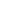true

# Free CBSE Class 7 Maths Worksheets

Download free printable Maths Worksheets to practice. With thousands of questions available, you can generate as many Maths Worksheets as you want.

## Sample CBSE Class 7 Maths Worksheet Questions

1.

3x(4+5) = (3x4) + (3x5). This is based on the ----- property over addition of integers.

1.

Commutative

2.

Distributive

3.

Associative

2.

Multiplicative Inverse (Reciprocal) of 5 is ----.

1.

1/5

2.

5

3.

0

4.

1

3.

(-3) ÷ (3) = ---- , but (-3) ÷ (-3) is = ----.

1.

-1, 1

2.

1,-1

3.

0,1

4.

1,0

4.

11+10 is = 21 and 10+11 is also = 21. The property used here is -----------.

1.

2.

3.

5.

Product of even number of negative integers is ----, but the product of odd number of negative integers is ------.

1.

Positive and Negative

2.

Negative and Positive

6.

The integer ---- is the identity under addition.

1.

1

2.

-1

3.

0

7.

3 + (2+1) = 2 + (1+3) = 1 + (2+3). This is based on the ---- property of integers.

1.

2.

3.

8.

Integers are closed for addition and subtraction, but not in ----- .

1.

Multiplication

2.

Division

9.

All whole numbers and natural numbers are also integers.

1. True 2. False
10.

The sum of a positive integer and its negative is equal to ---

1.

0

2.

-1

3.

1

4.

NA

## Find more Maths Worksheets

Worksheets by UrbanPro

Our worksheets are designed to help students explore various topics, practice skills and enrich their subject knowledge, to improve their academic performance. Designed by Experts who have extensive experience and expertise in teaching a subject, these worksheets will improve your child's problem-solving skills and subject knowledge in a fun and interactive manner.
Check out our free customized worksheets across school boards, grades, subjects and levels of subject knowledge. You can download, print and share these worksheets with anyone, anywhere, anytime!

Get a custom worksheet to practice!

Select your topic & see the magic.

subjectSelect Chapter(s)

Chapters & Subtopics